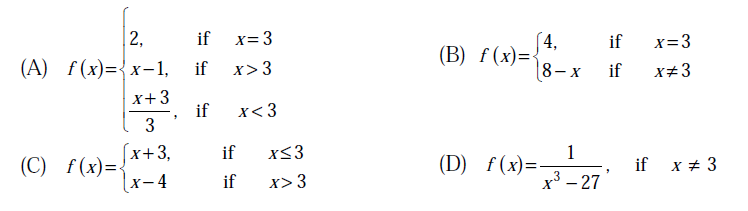# GATE | GATE CS 2013 | Question 22

Which one of the following functions is continuous at x = 3?(A) A
(B) B
(C) C
(D) D

Explanation: A function is continuous at some point c,

Value of f(x) defined for x > c = Value of f(x) defined for x < c = Value of f(x) defined for x = c

All values are 2 in option A

Quiz of this Question

My Personal Notes arrow_drop_up
Article Tags :

Be the First to upvote.

Please write to us at contribute@geeksforgeeks.org to report any issue with the above content.